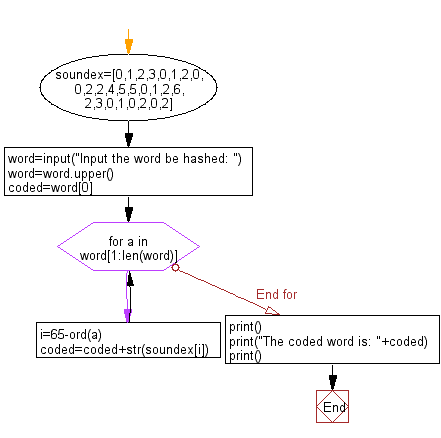﻿ Python: Hash a word - w3resource# Python: Hash a word

## Python Basic: Exercise-74 with Solution

Write a Python program to hash a word.

Sample Solution-1:

Python Code:

``````soundex=[0,1,2,3,0,1,2,0,0,2,2,4,5,5,0,1,2,6,2,3,0,1,0,2,0,2]

word=input("Input the word be hashed: ")

word=word.upper()

coded=word

for a in word[1:len(word)]:
i=65-ord(a)
coded=coded+str(soundex[i])
print()
print("The coded word is: "+coded)
print()
```
```

Sample Output:

```Input the word be hashed: w3r

The coded word is: W02
```

Flowchart:## Visualize Python code execution:

The following tool visualize what the computer is doing step-by-step as it executes the said program:

Sample Solution-2:

Python Code:

``````int_num = 112
flt_num = 23.99
text_val = 'Python Exercises'
print("Original integer value:",int_num)
print ("Hash value of the said integer value: " + str(hash(int_num)))
print("Original float value:",flt_num)
print ("Hash value of the said float value: " + str(hash(flt_num)))
print("Original text:",text_val)
print ("Hash value of the said text: " + str(hash(text_val)))
```
```

Sample Output:

```Original integer value: 112
Hash value of the said integer value: 112
Original float value: 23.99
Hash value of the said float value: 2282784579121553431
Original text: Python Exercises
Hash value of the said text: 8156633541458031964
```

## Visualize Python code execution:

The following tool visualize what the computer is doing step-by-step as it executes the said program:

Python Code Editor:

Have another way to solve this solution? Contribute your code (and comments) through Disqus.

What is the difficulty level of this exercise?

Test your Python skills with w3resource's quiz

﻿

## Python: Tips of the Day

List comprehension:

```>>> m = [x ** 2 for x in range(5)]
>>> m
[0, 1, 4, 9, 16]
```